• ### help to understang Ringmodulation

Hello everyone,
I have some question in Ring modulation
Can some body explain to me some theory in Ring modulation .
here is question.
Q: How come carrier Frequency phase cancel out in RM ?

For instance, if the carrier is 900 Hz and the modulator is 500 Hz, we will get two sidebands; one at 400 Hz (900 - 500) and one at 1400 Hz (900 + 500) and Carrier Frequency cancel out ?
How come ? There is no out of phase in this signal , then how do we get understand this ?
I was search on internet and could not find an answer.

last question in AM

suppose we use range -1 , 0, 1 amplitude.
there is two difference range of amplitude . one is -1,0,1other one is 0,1

a range of amplitude -1 ,0, 1 is longer than 0,1 rate because signal go through 0 , 1 ,-1 ,0, and 0,1 has only 0 to 1 and 0.
unipolar has short rate than bipolar.
but actually if i modulated two signal with those range of amplitude and compared.
the result was opposite.
bipolar is more fast than unipolar.
i think bipolar takes more time to hear the signal than unipolar
because the signal go through 0 and -1 , o to 1 that makes more time than unipolar.
I am curious how come range -1 , 0, 1 is fast than 0, 1 .
maybe i am completely lost this section.or completely wrong approach.
I really wanna understand this. can someone help me ?
lu.

• Posts 8 | Views 5131
• Hi and welcome to this forum,

I will try to address your first question (the second one is not very clear to me, you used a lot of terms that I do not know). I think that the best way to understand amplitude modulation (and ring modulation) is to realize that the output signal is dictated by the interaction of both input signals, not by themselves. In a certain way, it is the same as asking why when you multiply two numbers the result is different than both of them.

When you multiply two signals, they simply interact in a way that results in two frequencies that are not necessarily the same as their own. You can visually see this process in the images below. I created three arrays in Pure Data, one containing the resultant signal of RM between 500 and 900Hz. The second is a pure 400 Hz sine wave and the third is a pure 1400 Hz sine wave: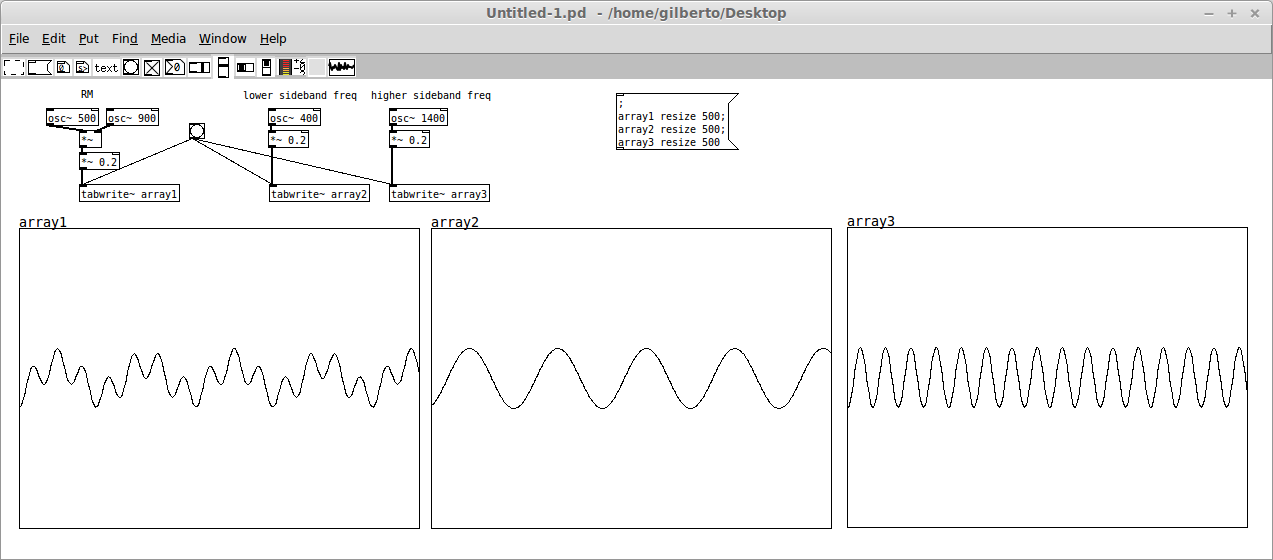Have a look what happens when I superimpose the result of RM with the 400 Hz: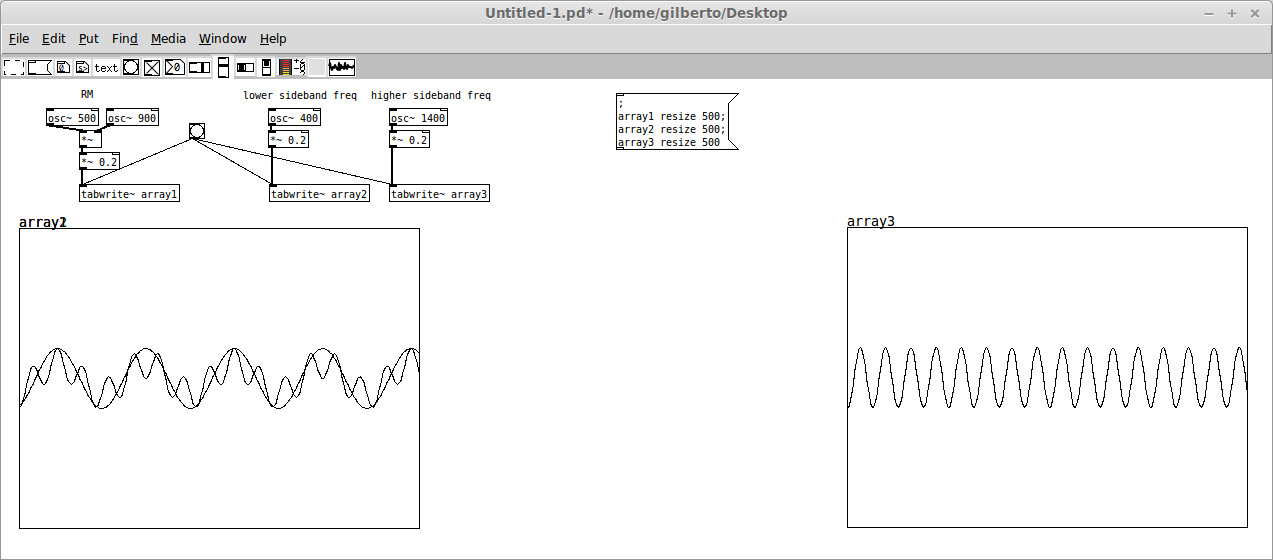And now when I superimpose it with the 1400Hz: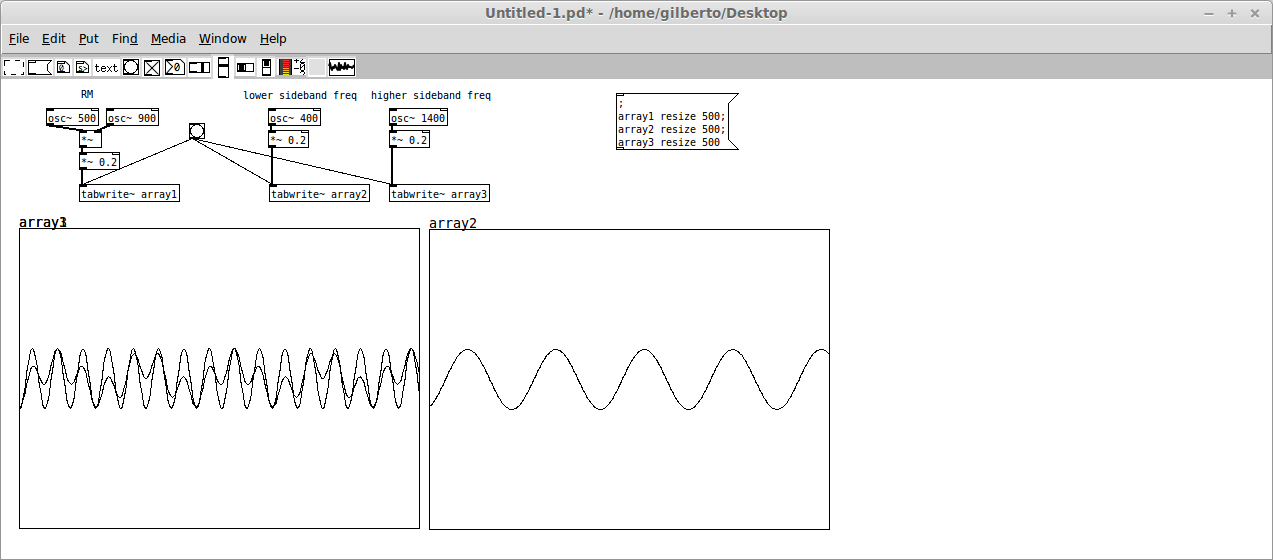Do you see how the peaks match? Can you visualize that that output contains only those two frequencies and nothing else?
I myself simply accept that this is the mathematical reality of multiplying waves.

You can also see this other example: what happens when you modulate 900 Hz with an LFO of 1 Hz? Acoustically sepaking, the result is a 900 Hz with a 2Hz tremolo (the tremolo is 2 x the LFO, since the LFO ranges from -1 to 1), right? But now let's see this from the points of view of two bands. RM between those two frequencies will result in 901 and 899 Hz, correct? And what happens when you have two frequencies this close being played together? You get it: one single frequency and a heavy beating: 900Hz with a 2hz tremolo.

I hope some of this will help you understand this subject a bit better.

Take care,
Gilberto

• Hello Gilberto,
thanks a lot for example and explain.
Many part are much more clear to me.
Some part a bit confused to me.
"LFO ranges from -1 to 1"
What I understand range -1 to 1 amplitude is full cycle of 1Hz , thus -1 to 1 = 1Hz.
or do i to think -1 is half and 1 is half thus 2Hz ?
i don't understand What makes 2Hz ?
Do i miss something before to understanding this concept ?

• Hi, my pleasure.

About your question, it is easier to visualize it than to explain it: below I have a 500 Hz wave being AM by an LFO of 1Hz. In the 2nd graph, I have a 1 Hz wave and in the 3rd graph another 1 Hz (but with a phase shifted by pi). Each graph spans for exactly 1 second. So: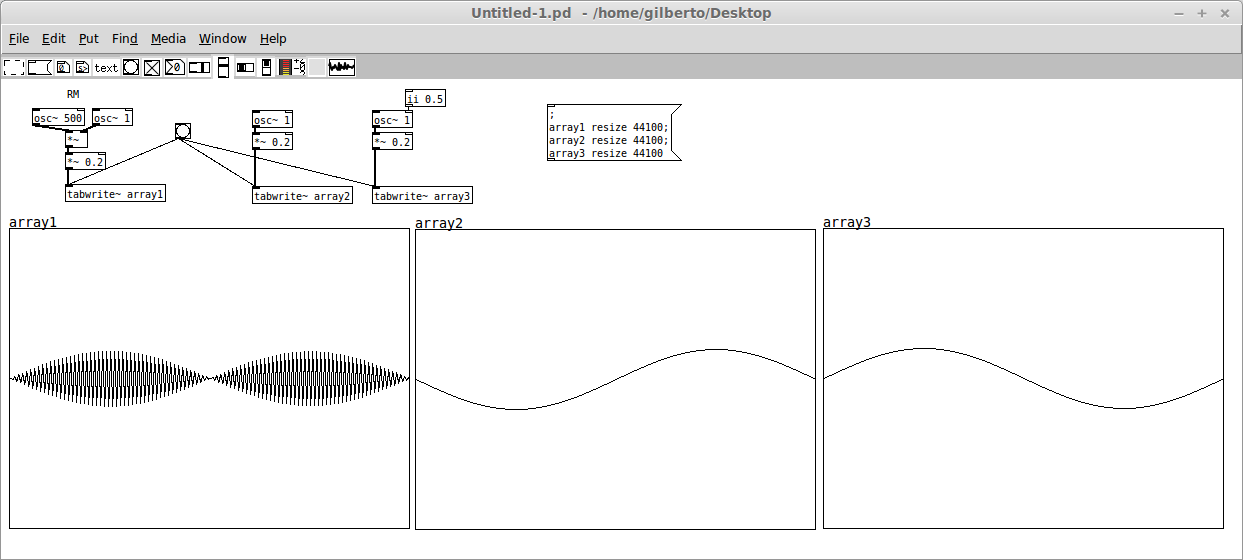Do you see how there are 2 tremolos as the result of the ring modulation in 1 second? That is, the tremolo frequency is being 2 Hz. It is as if the "contour" of the array 1 is given by the following: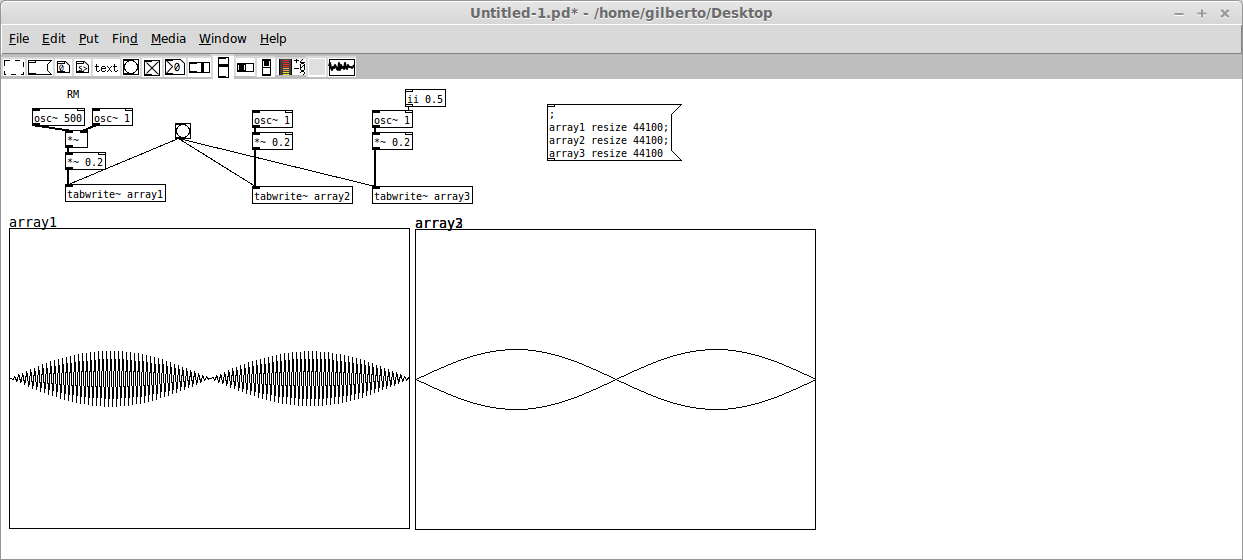Mathematically it is easier to understand: between 0 and 0.5 seconds, the LFO is negative, while between 0.5 until 1 s it is positive right? But in the end this value does not matter, since the carrier frequency is quickly oscillating between -1 and 1. So in the 1st half (0-0.5s), when the carrier frequency is negative the resultant signal is positive, and when the carrier is positive the resultant is negative. But since this oscillation is much quicker than the LFO itself, the result is that the contour looks mirrored. The sounding result is this two peaks per second.

I hope this helps. Take care,
Gilberto

• Hello Gilberto ,

Fantastic explanation.
I was spend many time today to understand this concept.
Finally You help me a lot to figured out.
Now I understand it. and Finally can get some sleep.

Thanks again

Cheers.

• Hi Luna, I am glad to be of any help. Good luck with your investigation!

• Here is the pyext build i made on my raspberry pi. If you don't want to compile pyext for raspberry by yourself, you could try it first.????

ID

• @TumB0 Hi and welcome to this forum,

For some reason your upload is not showing in your post. If you want to upload a file, you have to click in the icon with a little arrow up (5th icon) while writing or editing a message.

But I also did not understand how your post is related to ring modulation. If it is, please explain it better. If not, please create a new topic.

Cheers!
Gilberto

Posts 8 | Views 5131
Internal error.

Oops! Looks like something went wrong!